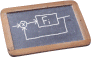# Automatic Control

COURSE DESCRIPTION

Part 1: (Master's course in Mechatronics Engineering + Undergraduate course in Electronics and Telecommunications Engineering)

Introduction: the control problem, examples of control applications. Continuous-time linear dynamical systems: ordinary differential equations, linear systems and state-space realization, solution and exponential matrix, natural and forced response, equilibria and stability, stability of linear systems, Laplace transform, transfer function, poles and zeros, DC-gain, dynamical models of physical systems (mechanical, electrical, hydraulic, thermal). Discrete-time linear systems: difference equations, discrete-time linear systems, solution method, stability, Zeta transform, transfer function. State-feedback control: reachability, controllability, stabilizability, pole placement via linear state feedback, integral action via state augmentation. State estimation: observability, reconstructability, detectability, duality, linear observer design. Output feedback control: static output feedback, dynamic compensator.

Part 2: (Master's course in Mechatronics Engineering)

Analysis and control design in the frequency domain: harmonic response, steady-state solution, Bode diagrams, Nyquist criterion, loop shaping, PID controller, block diagrams. Sampling: zero-order holder, exact sampling, digital implementation of continuous-time controllers, selection of sampling time. Anti-windup design: schemes for PID control, observer-based schemes. Advanced discrete-time techniques: deadbeat control, delay compensation, preview of reference and measured disturbance. Basics of modern control: optimal control and estimation (linear quadratic regulation, Kalman filtering), model reduction, system identification (ARX and ARMAX), nonlinear systems (Lyapunov stability, feedback linearization), model predictive control.

PREREQUISITES

Linear algebra and matrix computation, calculus and mathematical analysis, complex analysis, physics (classical mechanics), circuit analysis.

TIMETABLE

Every Tuesday, Wednesday, Thursday from 10.30 to 13.00 in room A102 (Povo 1 “Fabio Ferrari” building), starting March 1, 2011 until mid June 2011 (national holidays excluded). Possible variations of time-table and lecture room will be announced during the course.

LECTURE SLIDES

Part 1

Part 2

EXERCISES

Exercise collection (past written examinations)

2011

2010

A good set of exercises can be found also here (from my previous Digital Control course, in Italian)

Last update: June 15, 2014